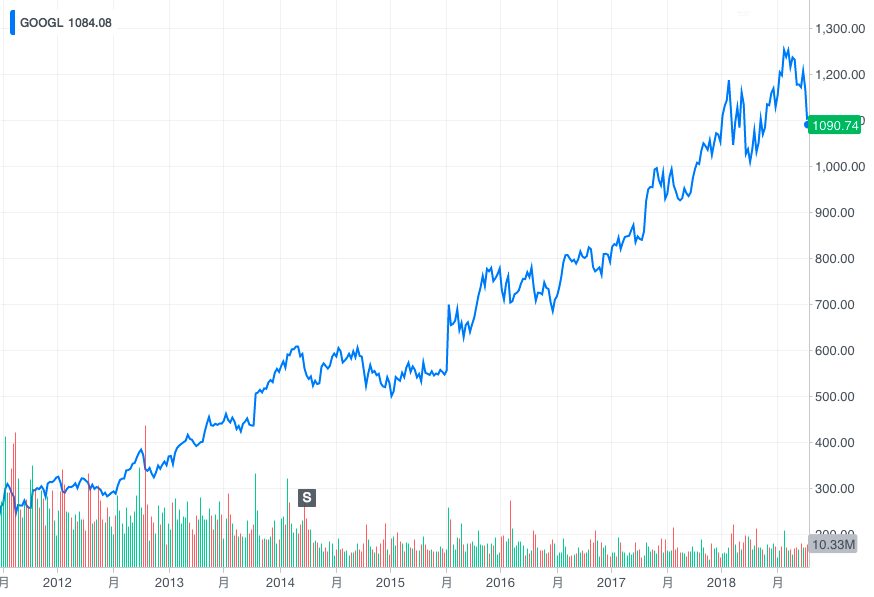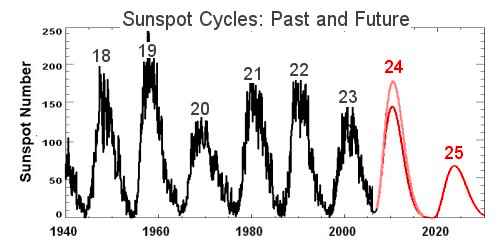## 数据分析：时间序列数据建模分析

6315 字 · 288 阅读 · 2023 年 06 月 17 日### 知识点

• 时序数据特点及分类
• 描述性时序分析
• 统计时序分析
• 平稳时间序列检验
• 自相关图和偏自相关图
• 纯随机性检验
• ARMA 介绍及建模
• 差分运算
• ARIMA 介绍及建模### 时序数据特点及分类

• 时间序列数据依赖于时间，但不一定是时间的严格函数。
• 时间序列数据每时刻上的值具有一定的随机性，不可能完全准确地用历史值去预测。

• 时间序列数据前后时刻（但不一定是相邻时刻）的数值往往具有相关性。
• 从整体上看，时间序列往往会呈现出某种趋势性或出现周期性变化的现象。

• 按研究对象分类：一元时间序列和多元时间序列。
• 按时间参数分类：离散时间序列和连续时间序列。
• 按统计特性分类：平稳时间序列和非平稳时间序列。
• 按分布规律分类：高斯型时间序列和非高斯型时间序列。

### 描述性时序分析### 平稳时间序列检验

import pandas as pd
from matplotlib import pyplot as plt
%matplotlib inline

"http://labfile.oss.aliyuncs.com/courses/1176/total-population.csv", index_col=0)
series1.plot(figsize=(9, 6))


import numpy as np

np.random.seed(10)  # 随机数种子
series2 = np.random.rand(1000)  # 生成随机序列
plt.plot(series2)  # 绘图
plt.ylim(5, -5)


### 自相关图

ACF 计算图示如下，原序列为延迟 $k$ 个时间间隔后计算与原序列之间的相关性系数（绿色部分）。Python 中，我们可以利用 statsmodels 统计计算库中的 plot_acf() 函数计算和绘制自相关图，也可以使用 Pandas 提供的 autocorrelation_plot() 方法绘制。

from statsmodels.graphics.tsaplots import plot_acf
from pandas.plotting import autocorrelation_plot

fig, axes = plt.subplots(ncols=2, nrows=1, figsize=(12, 4))

plot_acf(series1, ax=axes)
autocorrelation_plot(series1, ax=axes)


fig, axes = plt.subplots(ncols=2, nrows=1, figsize=(12, 4))

plot_acf(series2, ax=axes)
autocorrelation_plot(series2, ax=axes)


### 纯随机性检验

Python 中，我们可以利用 statsmodels 统计计算库中的 acorr_ljungbox() 函数计算 LB 统计量，该函数默认会返回 LB 统计量和 LB 统计量的 P 值。如果 LB 统计量的 P 值小于 0.05，我们则认为该序列为非随机序列，否则就为随机序列。

from statsmodels.sandbox.stats.diagnostic import acorr_ljungbox

LB2, P2 = acorr_ljungbox(series2)
plt.plot(P2)


series3 = pd.read_csv(
"http://labfile.oss.aliyuncs.com/courses/1176/sunspot.csv", index_col=0)
series3.index = pd.period_range("1820", "1869", freq='Y')  # 将索引转换为时间
series3.plot(figsize=(9, 6))


fig, axes = plt.subplots(ncols=2, nrows=1, figsize=(12, 4))

plot_acf(series3, ax=axes)
autocorrelation_plot(series3, ax=axes)


LB3, P3 = acorr_ljungbox(series3)
plt.plot(P3)


### ARMA 介绍及建模

ARMA 模型的全称是自回归移动平均模型，它是目前最常用的拟合平稳序列的模型。ARMA 模型一般又可以被细分为 AR 自回归模型，MA 移动平均模型和 ARMA 三类。

AR 模型非常简单，其思路来源于线性回归。即假设序列包含线性关系，然后使用 $x_{{1}}$ 至 ${x_{t-1}}$ 序列来预测 ${x_{t}}$。其中，$p$ 阶 AR 模型的公式为：

$$X_{t}=c+\sum _{{i=1}}^{p}\varphi _{i}X_{{t-i}}+\varepsilon _{t}$$

$${x_{t}=\varepsilon _{t} + \theta _{1}\varepsilon _{t-1} + \theta _{2}\varepsilon _{t-2} + \cdots + \theta _{q}\varepsilon _{t-q}}$$

ARMA 模型一般记作：$ARMA(p,q)$，即为 $p$ 阶 AR 和 $q$ 阶 MA 模型的组合。Python 中，我们可以利用 statsmodels 统计计算库中的 tsa.ARMA 类完成 ARMA 建模和预测。

import warnings
from statsmodels.tsa.stattools import arma_order_select_ic

train_data = series3[:-10]  # 80% 训练
test_data = series3[-10:]

warnings.filterwarnings('ignore')
arma_order_select_ic(train_data, ic='aic')['aic_min_order']  # AIC

arma_order_select_ic(train_data, ic='bic')['bic_min_order']  # BIC

arma_order_select_ic(train_data, ic='hqic')['hqic_min_order']  # HQIC


from statsmodels.tsa.arima_model import ARMA

arma = ARMA(train_data, order=(2, 1)).fit()  # 定义并训练模型


plt.plot(arma.forecast(steps=10), '.-', label="predict")  # 输出后续 10 个预测结果
plt.plot(test_data.values, '.-', label="real")
plt.legend()


### 差分运算

$$\nabla x_t = x_t - x_{t-1}$$

$$\nabla^2 x_t = \nabla x_t - \nabla x_{t-1}$$

$$\nabla^p x_t = \nabla^{p-1} x_t - \nabla^{p-1} x_{t-1}$$

$$\nabla_k x_t = x_t - x_{t-k}$$

fig, axes = plt.subplots(ncols=3, nrows=1, figsize=(15, 3))

diff1 = series1.diff().dropna()  # 1 阶差分

axes.plot(diff1)  # 绘图
autocorrelation_plot(diff1, ax=axes)  # 平稳性检验
axes.plot(acorr_ljungbox(diff1))  # 纯随机检验


fig, axes = plt.subplots(ncols=3, nrows=1, figsize=(15, 3))

diff1 = series1.diff(periods=2).dropna()  # 1 阶 2 步差分

axes.plot(diff1)  # 绘图
autocorrelation_plot(diff1, ax=axes)  # 平稳性检验
axes.plot(acorr_ljungbox(diff1))  # 纯随机检验


fig, axes = plt.subplots(ncols=3, nrows=1, figsize=(15, 3))

diff2 = series1.diff().diff().dropna()  # 2 阶差分

axes.plot(diff2)  # 绘图
autocorrelation_plot(diff2, ax=axes)  # 平稳性检验
axes.plot(acorr_ljungbox(diff2))  # 纯随机检验


### ARIMA 介绍及建模

train_data = series1[:-40]  # 约 80% 训练
test_data = series1[-40:]

arma_order_select_ic(train_data.diff().dropna(), ic='aic')['aic_min_order']  # AIC


from statsmodels.tsa.arima_model import ARIMA

arima = ARIMA(train_data, order=(2, 1, 2)).fit()  # 定义并训练模型


plt.plot(arima.forecast(steps=40), '.-', label="predict")  # 输出后续 40 个预测结果
plt.plot(test_data.values, '.-', label="real")
plt.legend()### 系列文章•